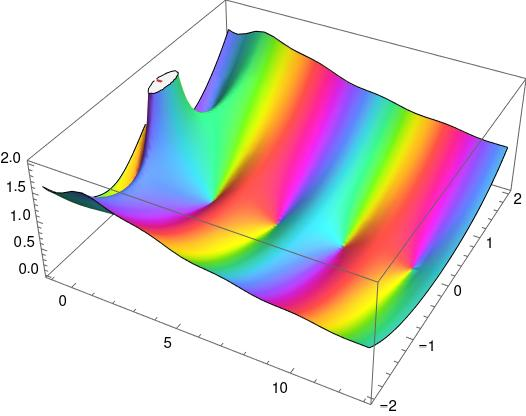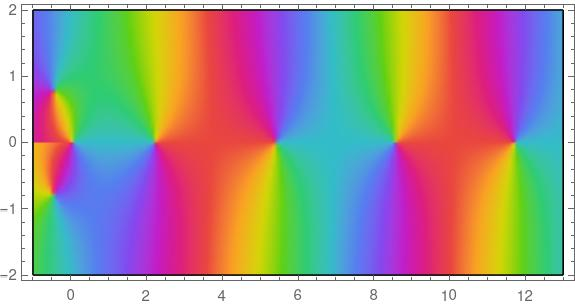# Sum the zeros of an analytic function without finding them first

A couple days ago I wrote about how Vieta’s formulas let you sum the zeros of a polynomial without having to first compute the zeros. This is especially handy for high-order polynomials since there is no explicit formula for the zeros.

Most functions that arise in applications are not polynomials. How could you find the sum of the zeros of an analytic function in some region without having to locate each of the zeros first? What if you don’t even know how many zeros are in the region?

Here’s a plot of the complex function we’re going to use as an example.As with yesterday’s post, the key is to use the argument principle, but this time a more general form.

## Generalized argument principle

Suppose we have a simple closed curve C and a function f(z) that is analytic in and on C. In particular we’re assuming f has no poles in or on C. Assume f has n zeros labeled z1 through zn. We’re going to integrate around C to add up the zeros of f inside.

Let g(z) be a function that we want to apply to the zeros of f. We assume it is also analytic in and on C. ThenIn words, we can sum the values of g applied to each of the zeros of f by computing the integral on the left.

In the previous post, we had g(z) = 1. That is, we were simply counting the zeros. To sum the zeros, we set g(z) = z.

## Example

The function plotted above is the Bessel function Y1. Here’s a flattened plot, just showing the phase of the values.The points along the real line where the colors swirl around a point are the zeros of the function. We can see that there are zeros near 2, 5, 9, and 11.

There’s something different going on at 0. The colors swirl in the opposite direction because there’s a pole at 0. And there’s an abrupt change in color on the real line to the left of 0 because there’s a branch cut there.

We want to find the sum of the first four zeros, so we’ll create a contour that includes these zeros and excludes the singularity at 0. We’ll use a rectangle with lower left corner at 1 – i and an upper right corner at 13 + i.

We’ll modify our Mathematica code from yesterday to use a different example function, Y1, and add a factor of z to the integrand to sum zeros rather than count zeros.

    f[z_] := BesselY[1, z]
leg[z1_, z2_] := NIntegrate[z f'[z]/f[z], {z, z1, z2}]
(leg[1-I, 13-I] + leg[13-I, 13+I] + leg[13+I, 1+I] + leg[1+I, 1-I])/(2 Pi I)


This returns 27.972 – 5.65432*10^-16 I. We know that the zeros are all real, so the imaginary part is integration error. We can ask Mathematica for more digits, and it will report the real part as 27.97198306597801.

We can compute the sum of the first four zeros directly

    N[Sum[BesselYZero[1, n], {n, 1, 4}]]

and the result agrees with the result above to 13 significant figures.

When we were counting zeros, we knew the result had to be an integer, so the rounded integral was exact. Here our result is only as accurate as our numerical integration, though we did use prior information to know that we could discard the imaginary part of the integration result. This would not have made any difference since the imaginary part is orders of magnitude smaller than the integration error in the real part.